Next: Conventional use of Bayes' Up: Conditional probability and Bayes' Previous: Conditional probability   Contents

## Bayes' theorem

Let us think of all the possible, mutually exclusive, hypotheses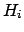which could condition the event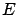. The problem here is the inverse of the previous one: what is the probability ofunder the hypothesis thathas occurred? For example, what is the probability that a charged particle which went in a certain direction and has lost between 100 and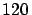keV in the detector is a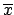, a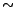, a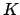, or a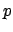?" Our eventis energy loss between 100 andkeV'', andare the four particle hypotheses''. This example sketches the basic problem for any kind of measurement: having observed an effect, to assess the probability of each of the causes which could have produced it. This intellectual process is called inference, and it will be discussed in Section.

In order to calculate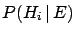let us rewrite the joint probability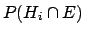, making use of (-), in two different ways: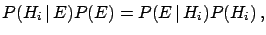(3.7)

obtaining(3.8)

or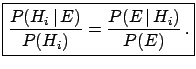(3.9)

Since the hypothesesare mutually exclusive (i.e.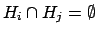,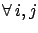) and exhaustive (i.e.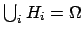),can be written as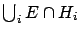, the union of the intersections ofwith each of the hypotheses. It follows that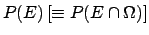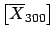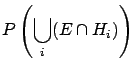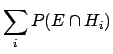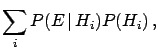(3.10)

where we have made use of () again in the last step. It is then possible to rewrite () as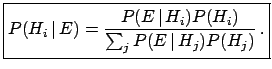(3.11)

This is the standard form by which Bayes' theorem is known. () and () are also different ways of writing it. As the denominator of () is nothing but a normalization factor, such that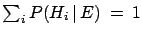, the formula () can be written as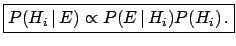(3.12)

Factorizing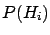in (), and explicitly writing that all the events were already conditioned by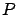, we can rewrite the formula as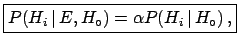(3.13)

with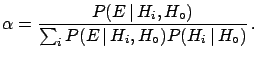(3.14)

These five ways of rewriting the same formula simply reflect the importance that we shall give to this simple theorem. They stress different aspects of the same concept.
To better understand the terms initial'', final'' and likelihood'', let us formulate the problem in a way closer to the physicist's mentality, referring to causes and effects: the causes could be all the physical sources which may produce a certain observable (the effect). The likelihoods are -- as the word says -- the likelihoods that the effect follows from each of the causes. Using our example of the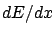measurement again, the causes are all the possible charged particles which can pass through the detector; the effect is the amount of observed ionization; the likelihoods are the probabilities that each of the particles give that amount of ionization. Note that in this example we have fixed all the other sources of influence: physics process, HERA running conditions, gas mixture, high voltage, track direction, etc. This is our. The problem immediately gets rather complicated (all real cases, apart from tossing coins and dice, are complicated!). The real inference would be of the kind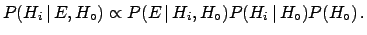(3.15)

For each state(the set of all the possible values of the influence parameters) one gets a different result for the final probability3.8. So, instead of getting a single number for the final probability we have a distribution of values. This spread will result in a large uncertainty of. This is what every physicist knows: if the calibration constants of the detector and the physics process are not under control, the systematic errors'' are large and the result is of poor quality.Next: Conventional use of Bayes' Up: Conditional probability and Bayes' Previous: Conditional probability   Contents
Giulio D'Agostini 2003-05-15